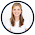Easter Egg Math Practice

Plastic Easter eggs and number cards.  That's it.  That's all you need for some super easy seasonal math practice.  Throw in a small white board and dry erase marker and you have an easy math center or small group activity ready to go.  And the beauty of Easter Egg Math is that it can work for a variety of levels - from basic number sense to addition and subtraction to multiples and rounding numbers.  There is something for everyone.

To get started, you will need number cards to put inside the Easter eggs.  I just cut a small index card into 8 pieces but you could probably go even smaller or larger and fold it so it will fit in the egg.  Then write the numbers that your students need to work on - from basic one digit numbers to 5 or 6 digit numbers.  Differentiate this so it is exactly what your students need.  If you have kids working on different skills that need different numbers, just color code them by using certain color eggs for each group of students.

Once you have your eggs filled with number cards, you are ready to check out some of these simple math activities from preschool through upper elementary.

Preschool/Kindergarten

Number match

Have duplicates of the numbers and have students open the eggs in search of matching numbers.

Draw a number

Pick a number.  Draw that amount of objects (of the student's choice) on the board.

Write the number

Simply copy the number that is on the card so young learners practice forming numbers.  Say the number and then count to that number.

Count on from a number

Start at the number chosen.  Count & write the next five numbers in the sequence without having to start at 1.

Compare two numbers

Pick two numbers.  Write them both down drawing a picture if necessary.  Circle the one that has more.  Do it again and look for the one that has less.

Add or subtract within 5

Using just the numbers 0-5, practice basic addition and subtraction problems.

What makes 10

Use the numbers 1-9.  Pick a number and write it down.  Figure out how many more to make 10.

Lower ElementarySequencing numbers

Have students pick 5-7 numbers and then put those numbers in order from least to greatest or greatest to least.

Pick two numbers.  Write them on the board and then add them together.

Subtracting Numbers

Pick two numbers.  Write them on the board with the largest number first and subtract.

Create a story problem

Pick two numbers and create a story problem to solve.  It could be an addition or subtraction problem for example.  It must use both numbers in the problem somehow.

Compare two numbers

Pick two numbers and write them down.  Use <, > or = to show the relationship between the two numbers.

10 more, 10 less

Pick a number.  Write what 10 more and 10 less of that number would be.

Write in word form, expanded form, base ten blocks

Write the number down and then make that number three different ways - word form, expanded form and using base ten blocks.

Upper Elementary

Multiplying Numbers

Pick two numbers.  Write the numbers down and solve as a multiplication problem.

Dividing Numbers

Pick two numbers.  Write the numbers down and solve as a division problem.

Multiples of a number

Pick a number and write down the next 5 multiples of that number.

Create a story problem

Pick two numbers and write a story problem that can be solved using addition, subtraction, multiplication or division and uses those two numbers in it.

Write in word form, expanded form, base ten blocks

Pick a number and write it on the board.  Then make that number using base ten blocks.  Write it in word form and then expanded form.

Compare two numbers

Pick two numbers with 3 or more digits and write them down.  Use <, >, = to make the number sentence make sense.

Round numbers

Pick a number and round it to the nearest 100 or 10.

Super simple right?  That's what we need sometimes.  You don't always have time to create some complex activity.  But this gives students great practice to build their number sense and computation skills and it is fun because they get choice over what eggs they open. How are you going to use eggs for math?

This post is also featured on the TpT blog, check it out HERE.

1.1.